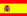You are here : > Home> Utilities> Economic and financial analysis  Sum of Years Digits Depreciation>

### Sum of digits depreciation

To calculate the annual depreciation of an asset by the sum of digits method, enter: Asset historical cost or initial cost of depreciable asset and number of years to depreciate, in accordance with legal regulations.
Use the point as decimal separator. Ex. For the number 25,352.43 enter 25352.43; The results will be displayed automatically after click on "Calculate".

 Sum of digits depreciation Depreciable asset value: Number of years: Depreciation Table